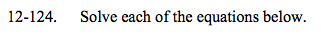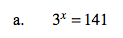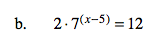### Home > A2C > Chapter 12 > Lesson 12.3.1 > Problem12-124

12-124.
1. Solve each of the equations below. Homework Help ✎

1. 3x = 141

2. 2 · 7(x −5) = 12Use properties of logarithms to solve.

x · log3 = log141

x ≈ 4.505Simplify, then see part (a).